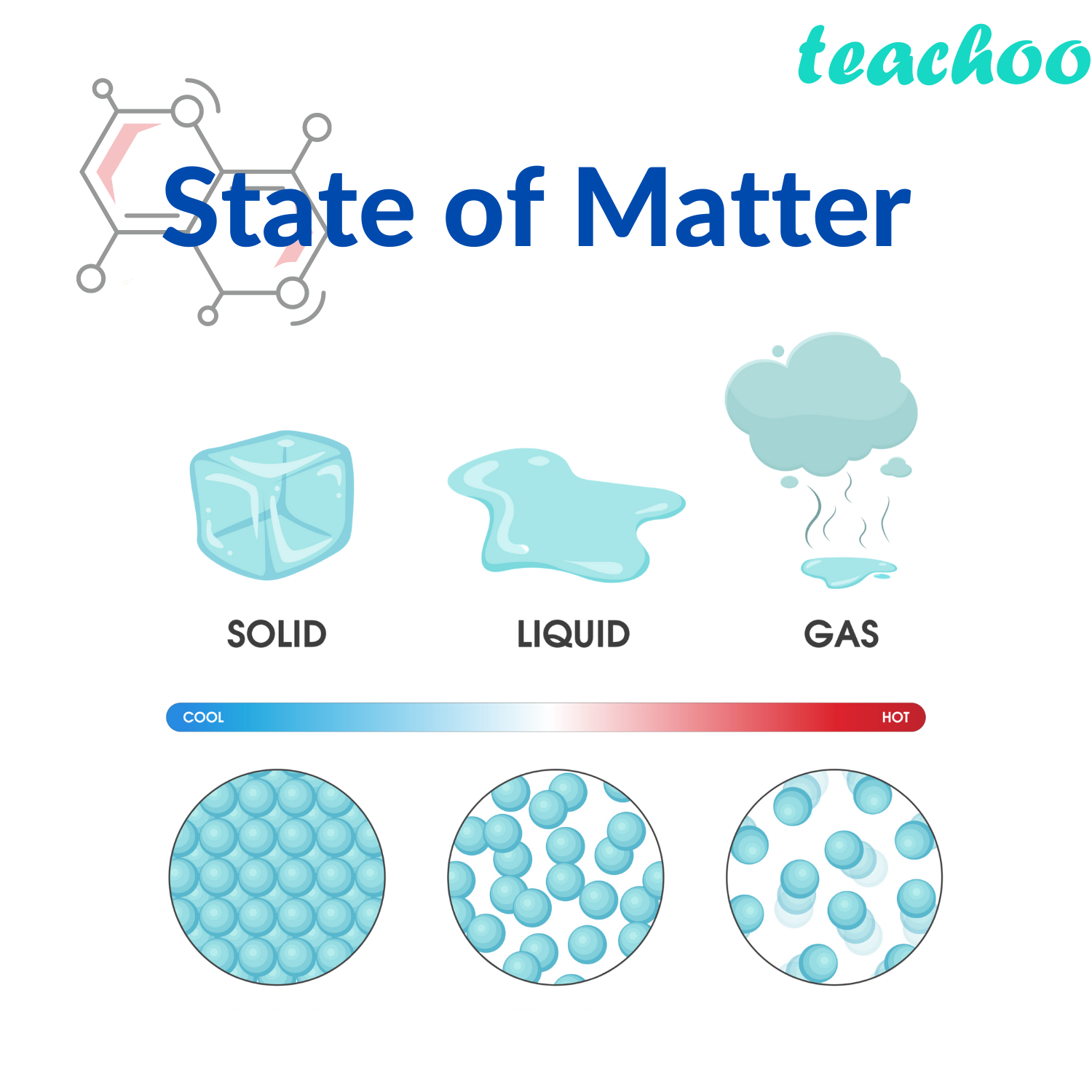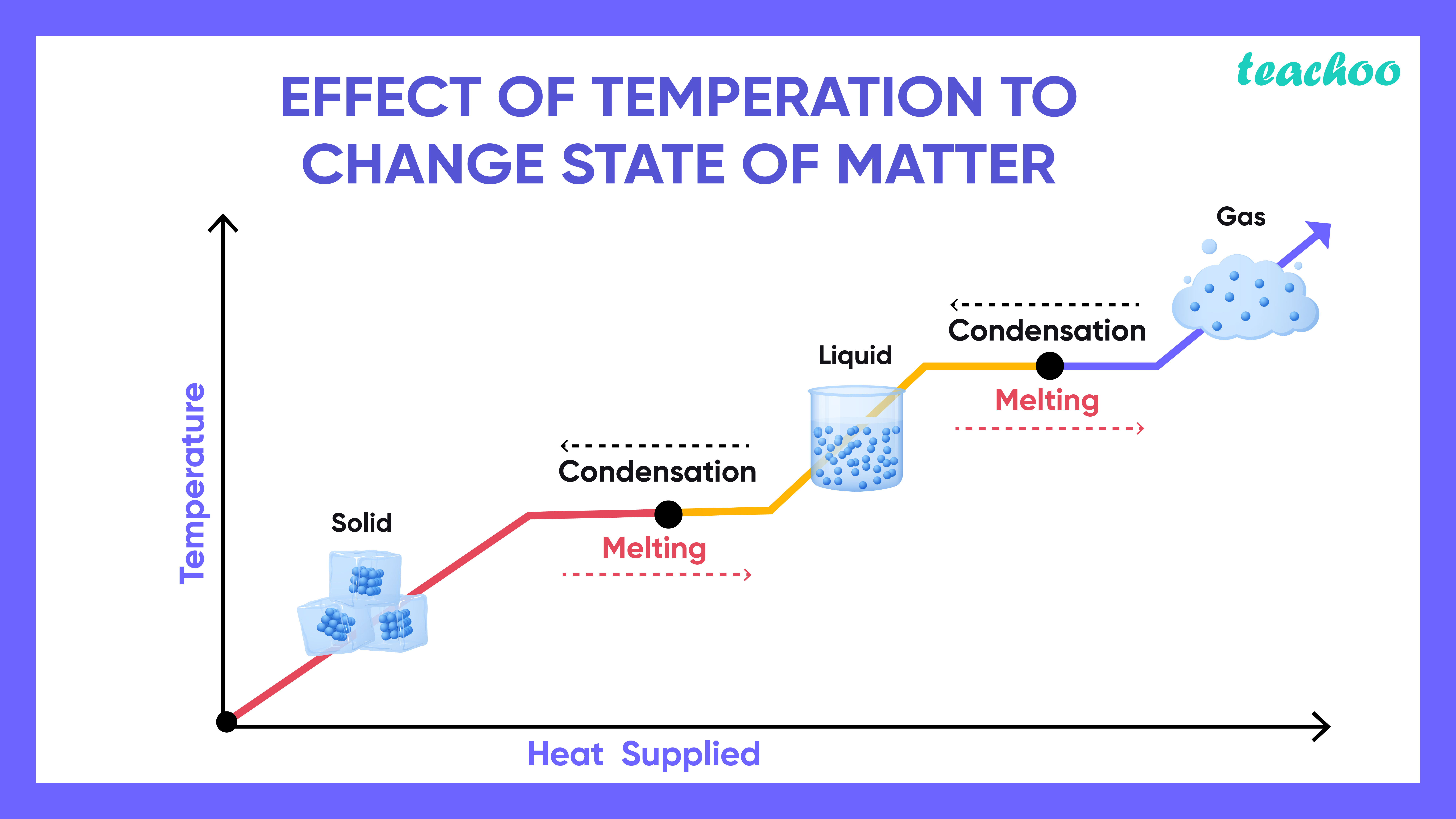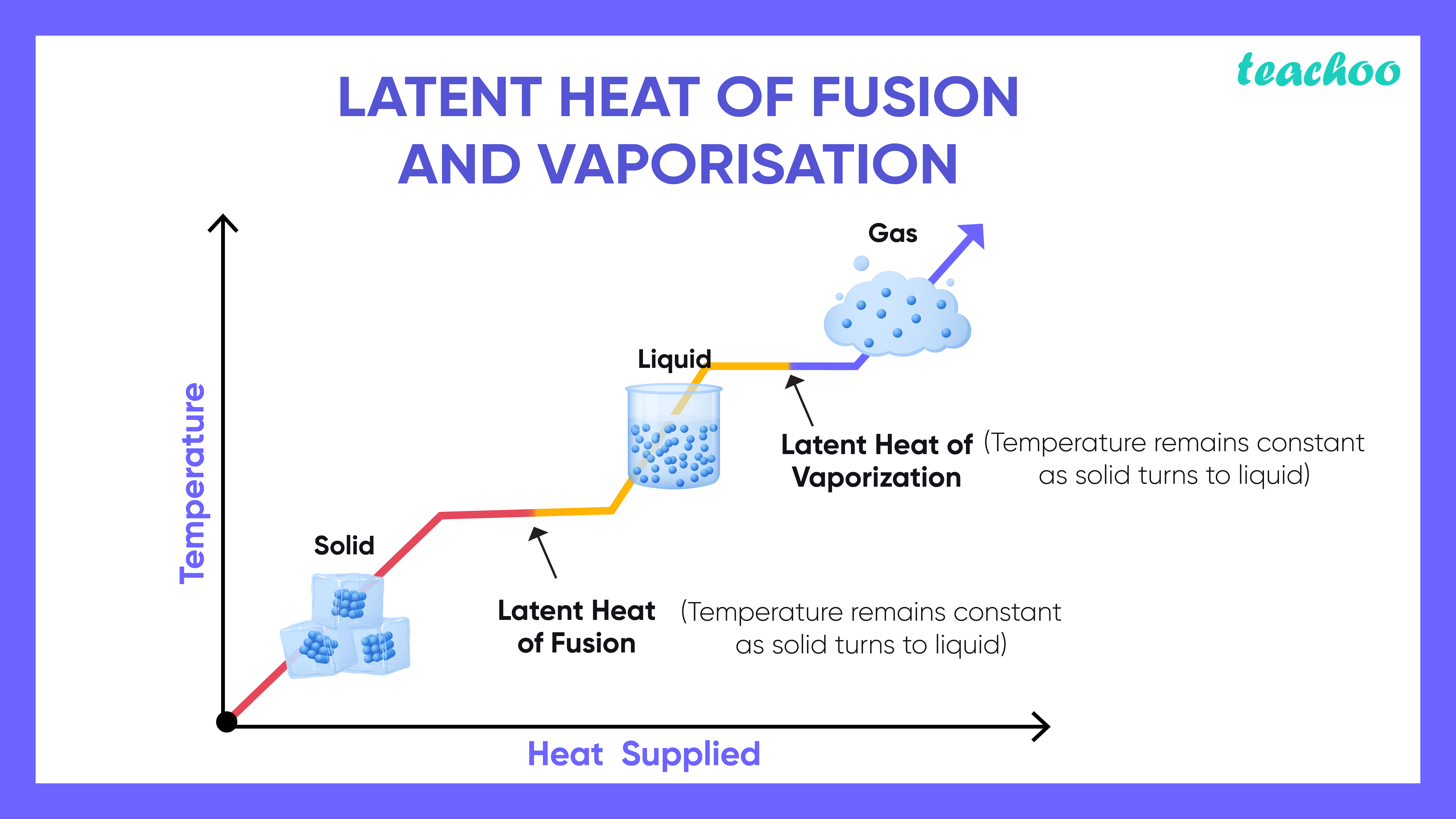Concepts

Class 9
Chapter 1 Class 9 - Matter In Our Surroundings

## Temperature

Temperature is the degree of hotness or coldness of a body.

It refers to the degree of heat present in a matter.

When we add heat or thermal energy to a substance, the temperature increases

• The kinetic energy of the particles increases and the particles vibrate faster
• The particles thus overcome the force of attraction between each other.
• Solids → Liquids → Gas (with increase in temperature)

The reverse happens when a substance in cooled

• The particles come closer to each other
• Gas → Liquid → Solid (with decrease in temperature)

## What happens if we heat a solid substance?

When we heat a solid substance, its temperature increases

This causes solid to melt (Convert into liquid)

Example

• If we take out ice from refrigerator and we keep it at room temperature
• It melts to water (due to an increase in temperature)## What causes this melting of solid?

• In solids, particles are closely bound together (due to high force of attraction between particles)
• But when we increase the temperature, the kinetic energy of particles increases
• This causes particles to move freely and overcome the force of attraction of particles
• Hence, solid melts into liquid

## What is the Melting Point of Solids?

The minimum temperature at which solid melts into liquids is called Melting Point of Solids

It indicates the strength of the force of attraction of different particles

Example

• Melting Point of Ice is 273.15 K (Kelvin) or 0 Degree Celsius
• Melting Point of Aluminium is 932K or 659 Celsius

## How to Convert Celsius into Kelvin?

• 0 Celsius = 273 Kelvin
• 1 Celsius = 273 + 1 = 274 K
• 100 Celsius = 273 + 100 = 373 K
• 659 Celsius = 273 + 659 = 932 K

## How does Temperature of Solid Change on Applying heat?

• When we apply heat on solid
• First, its temperature keeps on increasing
• When it reaches Melting Point, it starts converting into Liquid
• During this period, the temperature of solid does not change
• The temperature remains constant till the time all the solid melts into liquid due to latent heat of solid
• After the solid melts, its temperature begins to increase again## What is Latent Heat?

Heat Energy applied to change the state of a substance (Example from solid to liquid) is called Latent Heat

Explanation

Normally, when we apply heat on a substance, its temperature increases

However when we apply it during its change of state (example melting of solid to liquid)

Its temperature does not increase during a period when the whole of solid melts

it is because heat is utilized in overcoming the force of attraction of particles of a substance. Hence, it does not cause any heat

The word latent means hidden
Hence latent heat means heat which is hidden in beaker and does not cause any increase in temperature## What are Different Types of Latent Heat?

It is of 2 types

• Latent Heat of Fusion
• Latent Heat of Vapourization

### Latent Heat of Fusion

It is the amount of heat energy required to convert 1 kg of solid into a liquid state at the melting point

Example

• Latent heat of fusion of ice is 3.34 × 105 J/kg at 0 degree Celsius
• It means that 3.34 × 10 5 Joules of Heat is used to convert 1 kg of ice into the water at 0 degree Celsius

### Difference between Fusion and Solidification

 Fusion Solidification Conversion of Solid into Liquid is called Fusion Conversion of Liquid into Solid is called Solidification Latent Heat of Fusion is Used in this Process Latent Heat of Fusion is Released in this Process

### Latent Heat of Vapourization

It is the amount of heat energy required to convert 1 kg of liquid into vapour at boiling point

Example

• Latent heat of Vapourization of water is 22.5 × 10 5 J/kg at 100 degree Celsius
• It means that 22.5 × 10 5 Joules of Heat is used to convert 1 kg of ice into water at 0 degree Celsius

## Difference between Vapourization and Evaporation

 Vapourization Evaporation Conversion of Liquid into Gas is called Evaporation Conversion of Gas into Liquid State is called Evaporation Latent Heat of Vapourization is Used in this Process Latent Heat of Vapourization is Released in this Process

## What are Sublimation and Deposition?

### Sublimation

It means the conversion of Solids into Gas without conversion into Liquid State

Example

• Dry ice (Solid Carbon dioxide) converts into Gas(Carbon dioxide) at Room Temperature
• Naphthalene balls disappear( change into the gaseous state) without leaving any solids

### Deposition

It means Conversion of Gas to Solid without conversion into liquid is called Deposition

Example

In Winters, Ice accumulates on Window panes (This is because Water vapour-Gas converts into ice-Solid)

Learn in your speed, with individual attention - Teachoo Maths 1-on-1 Class